Next: The new R Up: Rosales: Damping Previous: Controlling the angle

# Numerical Examples

Figure 2 shows from left to right: a two-spike synthetic model, modeling with equations (1) and (2). It is possible to observe the decay of amplitude (energy) with respect to time when we include the damping factor () in our modeling equation. The same effect is observable in Figure 3, which shows the migration results using the results of Figure 2.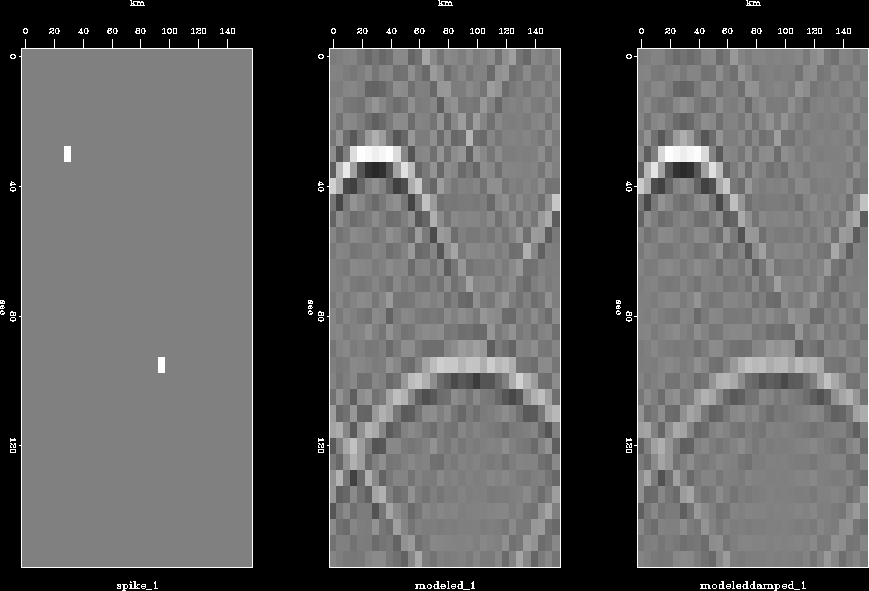compar_1
Figure 2
Modeling Comparison. From left to right: synthetic model; modeling with; modeling with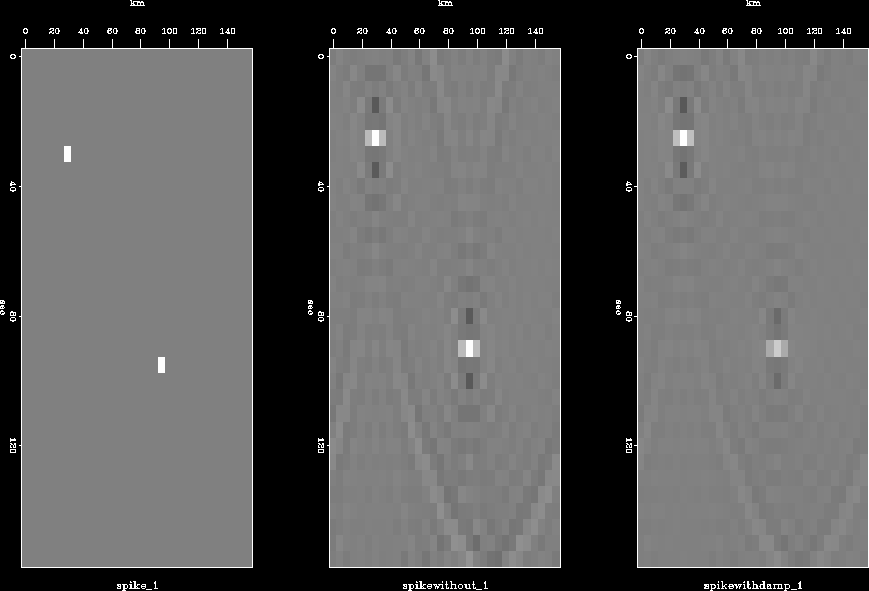modmig_1
Figure 3
Modeling-Migration Comparison. From left to right: synthetic model; results with;results withThe energy decay is a function of the damping factor (). Figure 4 presents experimental results of the energy variability with respect to.The experiment consists of modeling and migration of the two-spike model with equation (2) for different values of.Each sample in the plot (Figure 4) represents the energy of each modeling-migration result forranging between 0.002 and 0.04 every 0.002. It is possible to observe that the energy decays exponentially with respect to the damping factor; this decrease is due to the omission of causality in the redefinition of ikz.

 energy Figure 4 Energy decay with respect to the damping factor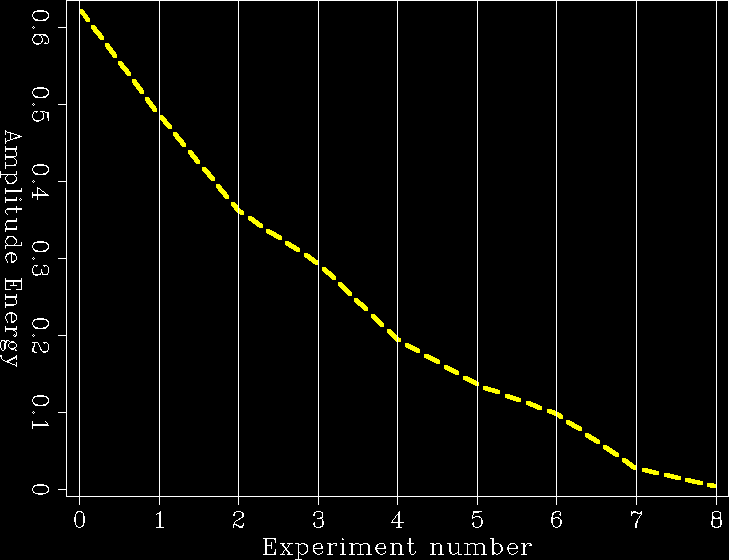For comparison, Figure 5 shows three results of the experimental procedure described before. It is possible to observe that the bigger the damping factor, the less energy is present in the final result.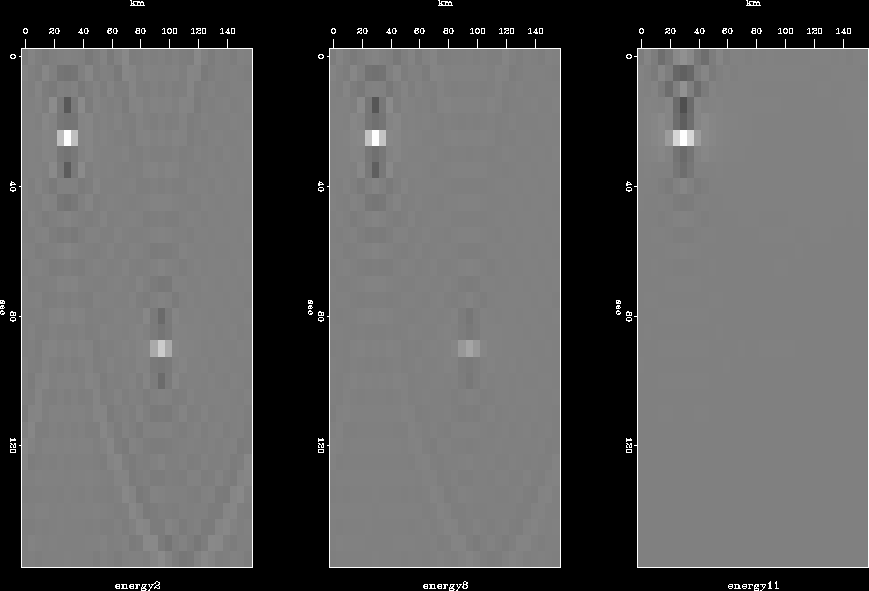threeep
Figure 5
Three different values offor the original model, right with, center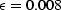, leftNext: The new R Up: Rosales: Damping Previous: Controlling the angle
Stanford Exploration Project
4/29/2001